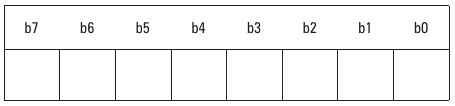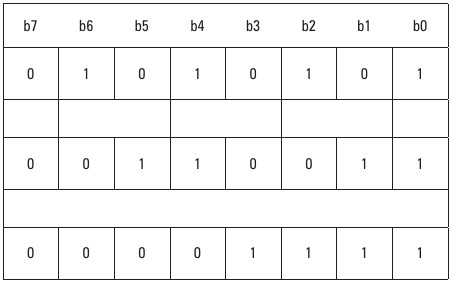# Power Programming: Bitwise Tips and Tricks

5
5858AdvertisementIf you are a seasoned programmer, these tips and tricks will seem very familiar, and are probably already part of your repertoire. If you are a novice programmer or a student, they should help you experience an “Aha!” moment. Independent of what you currently do, these tips and tricks will remind you of the wonderful discoveries in computer science, and the brilliant men and women behind them.

Before we get started, let’s establish some conventions for the rest of the article. Figure 1 shows how we represent bits — we start from right to left. The rightmost bit is the “Least Significant Bit” (LSB), and labelled as b0. The “Most Significant Bit” (MSB) is labelled b7. We use 8 bits to indicate and demonstrate concepts. The concept, however, is generically applicable to 32, 64, and even more bits.Figure 1: Typical bit-wise representation

## Population count

Population count refers to the number of bits that are set to 1. Typical uses of population counting are:

• Single-bit parity generation and detection: To generate the correct parity bit, depending on the scheme being followed (odd or even parity), one would need to count the number of bits set to 1, and generate the corresponding bit for parity. Similarly, to check the parity of a block of bits, we would need to check the number of 1s, and validate the block against the expected parity setting.
• Hamming weight: Hamming weight is used in several fields, ranging from cryptography to information theory. The hamming distance between two strings A and B can be computed as the hamming weight of “A” XOR “B”.
Advertisement

These are just a few of the use cases of population counting; we cannot hope to cover all possible use cases, but rather, just explore a few samples.

## First implementation

Our first implementation is the most straightforward:

```int count_ones(int num)
{
int count = 0;
int mask = 0x1;
while (num) {
if (num & mask)
count++;
num >>= 1;
}
return count;
}```

The code creates a mask bit, which is the number 1. The number is then shifted right, one at a time, and checked to see if the bit just shifted to the rightmost bit (LSB), is set. If so, the count is incremented. This technique is rather rudimentary, and has a cost complexity of O(n), where n is the number of bits in the block under consideration.

## Improving the algorithm

For those familiar with design techniques like divide and conquer, the idea below is a classical trick called the “Gillies-Miller method for sideways addition”. This process is shown in Figure 2.Figure 2: Divide and conquer the summation of bits
As the name suggests, this method involves splitting the bits to count; we start by pairing adjacent bits, and summing them. The trick, though, is that we store the intermediate result in the same location as the original number, without destroying the data required in the next step. The code for the procedure is shown below:

```static inline unsigned char bit_count(unsigned char x)
{
x = (0x55 & x) + (0x55 & (x >> 1));
x = (0x33 & x) + (0x33 & (x >> 2));
x = (0x0f & x) + (0x0f & (x >> 4));
return x;
}```

The key points to note for this algorithm are:

• It uses a mask at each step in the algorithm.
• The code takes O(log n) time to complete.

The masks at each step are shown in Figure 3.Figure 3: Masks to divide and conquer summation
The masks in the first step have alternate bits set (0x55); this selects alternate bits for summation. The number above is summed with the same mask (0x55), but with the entire number shifted right by one bit. In effect, this sums the alternate bits of the word. The bits that are not important are cleared, and set to 0 in the mask.

This procedure is repeated; the goal now is to compute the sum of the intermediate result obtained in the step above. The previous step counted the sum of alternate bits; it is now time to sum two bits at a time. The corresponding mask for this step is 0x33 (can you see why?). Again, we repeat the procedure by masking the number with 0x33, and adding to it the result of the number right-shifted by 2 and masked by 0x33. We do something similar in the final step, where we need to count 4 bits at a time, and sum up the result to obtain the final answer.

Figure 2 shows a sample computation for the number 177, which is represented as 10110001.

In Step 1, we sum the adjacent bits, leading to 01100001 (the sum of 1 and 0 is 01, the sum of 1 and 1 is 10 (in binary, this represents 2), the sum of 0 and 0 is 00, the sum of 0 and 1 is 01). In the next step, we sum 2 bits at a time, resulting in 00110001 (the sum of 01 and 10 is 0011 — 3 in binary; the sum of 00 and 01 is 0001).

In the final step, we sum 4 bits at a time, resulting in 00000100 (the sum of 0011 and 0001 is 00000100 — 4 in binary). As expected, this is also the final outcome, and the result of the number of 1s in the block under consideration.

This completes the sideways addition algorithm. As you can see, this algorithm is clearly more efficient than the initial approach.

## Exercises

1. We focused on 8 bits in a block to explain the algorithm. This algorithm can easily be extended to 32 or 64 bits and beyond. Write a routine to extend this algorithm to 64 bits, and potentially all the way up to 256 bits.
2. The algorithm specified above (in the section Improving the algorithm) is not necessarily optimal. Look at the references below to see if a more optimal version can be found and used. Explain what optimisations are possible, and how.
##### References
• MMIXware: A RISC Computer for the Third Millennium by Donald E Knuth, Springer-Verlag, 1999
• Matters Computational: ideas, algorithms, source code by Jorg Arndt, Draft version of 20-June-2010

This article was originally published in September 2010 issue of the print magazine.

Advertisement

#### 5 COMMENTS

1.JKR

More efficient? Probably. More readable? Definitely not.

2.Priya

Enjoyed the logic.. good work!

3. This will work for only 8bit data? (unsigned char?) – in that case if speed is the main criteria – I will go for look-up table. at the most I will waste 256 bytes of memory, but it will be super fast.